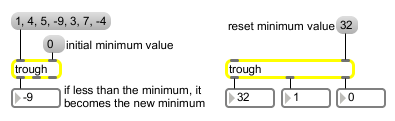# trough

If a number is less than previous numbers, output it

## Description

trough takes a number in the left inlet, compares to the old trough and, if it's smaller than the old trough, sends that number to the output and sets it as the new trough.

## Arguments

Name Type Opt Description
number-format float opt The initial value stored in trough is 128. Providing a float argument will cause trough to operate on floating-point values instead of integers.

## Messages

 bang In left inlet: Sends the currently stored minimum value out the left outlet. int input [int] In left inlet: If the input is less than the value currently stored in trough, it is stored as the new minimum value and is sent out. (inlet1) set-current-trough [int] In right inlet: The input is stored in trough as the new minimum value, and is sent out. float input [float] In left inlet: If the input is less than the value currently stored in trough, it is stored as the new minimum value and is sent out. (inlet1) set-current-trough [float] In right inlet: The input is stored in trough as the new minimum value, and is sent out.

## Output

int: Out left outlet: New minimum values are sent out. (Numbers received in the right inlet are always the new minimum value.)

Out middle outlet: If the number received is a new minimum value, the output is 1. If the number received in the left inlet is not a new minimum value, the output is 0.

Out right outlet: If the number received is a new minimum value, the output is 0. If the number received in the left inlet is not a new minimum value, the output is 1.

## ExamplesFind the smallest in a series of numbers... Number in right inlet always sets a new trough